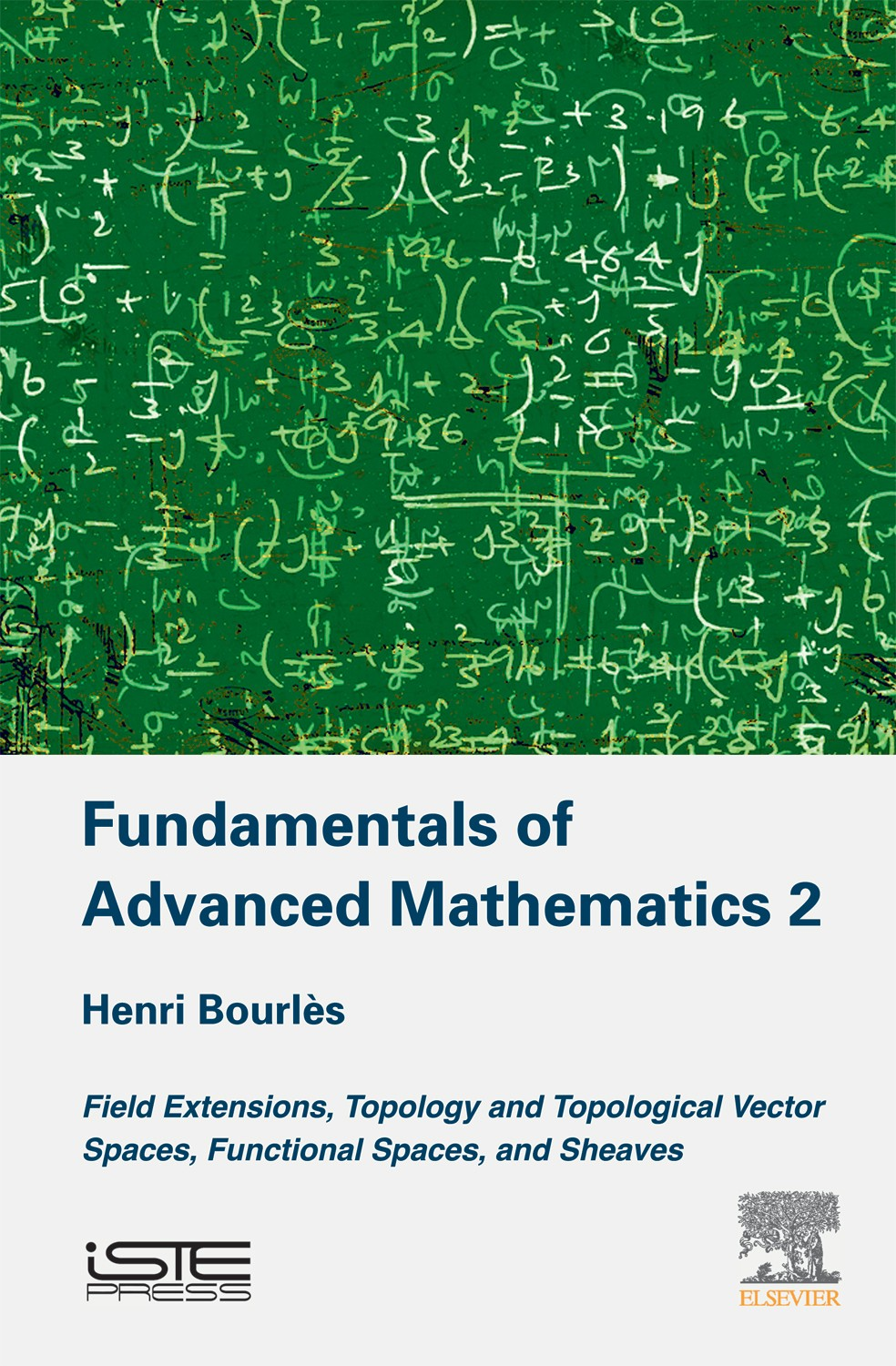pages
360
ISBN
9781785482496

The three volumes of this series of books, of which this is the second, put forward the mathematical elements that make up the foundations of a number of contemporary scientific methods: modern theory on systems, physics and engineering. Whereas the first volume focused on the formal conditions for systems of linear equations (in particular of […]

Disponible sur Amazon

The three volumes of this series of books, of which this is the second, put forward the mathematical elements that make up the foundations of a number of contemporary scientific methods: modern theory on systems, physics and engineering.

Whereas the first volume focused on the formal conditions for systems of linear equations (in particular of linear differential equations) to have solutions, this book presents the approaches to finding solutions to polynomial equations and to systems of linear differential equations with varying coefficients.

The book begins in Chapter 1 with Galois theory (both usual and differential). Then, the theoretical foundations are laid, starting by exploring topology, which is presented in Chapter 2. Subsequent chapters present different theories of advanced mathematics: topological vector spaces and their duals, functions of a single complex variables and generalized function spaces, including hyperfunctions of a single variable. Sheaves are discussed near the end of the book, where the author considers two specific applications: meromorphic functions and hyperfunctions, both in several variables.

These generalized functions are used to solve systems of linear differential equations with varying coefficients, leading to a result that is analogous to the result of algebraic analysis obtained in Volume 1 for systems of linear differential equations with constant coefficients.

1. Field Extensions and Differential Field Extensions.
2. General Topology.
3. Topological Vector Spaces.
4. Measure and Integration, Function Spaces.
5. Sheaves.

## Henri Bourlès

Henri Bourlès est professeur titulaire de chaire au Conservatoire national des arts et métiers. Il enseigne l’automatique dans des écoles d’ingénieurs et à l’université depuis plus d’une trentaine d’années. Ses travaux de recherche portent sur la théorie des systèmes.